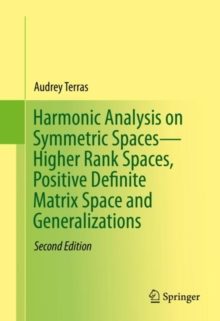Supporting your high street Find out how »
• My Account# Harmonic Analysis on Symmetric Spaces-Higher Rank Spaces, Positive Definite Matrix Space and Generalizations Hardback

#### Description

This text is an introduction to harmonic analysis on symmetric spaces, focusing on advanced topics such as higher rank spaces, positive definite matrix space and generalizations.

It is intended for beginning graduate students in mathematics or researchers in physics or engineering.

As with the introductory book entitled "Harmonic Analysis on Symmetric Spaces - Euclidean Space, the Sphere, and the Poincare Upper Half Plane, the style is informal with an emphasis on motivation, concrete examples, history, and applications.

The symmetric spaces considered here are quotients X=G/K, where G is a non-compact real Lie group, such as the general linear group GL(n,P) of all n x n non-singular real matrices, and K=O(n), the maximal compact subgroup of orthogonal matrices.

Other examples are Siegel's upper half "plane" and the quaternionic upper half "plane".

In the case of the general linear group, one can identify X with the space Pn of n x n positive definite symmetric matrices. Many corrections and updates have been incorporated in this new edition.

Updates include discussions of random matrix theory and quantum chaos, as well as recent research on modular forms and their corresponding L-functions in higher rank. Many applications have been added, such as the solution of the heat equation on Pn, the central limit theorem of Donald St. P. Richards for Pn, results on densest lattice packing of spheres in Euclidean space, and GL(n)-analogs of the Weyl law for eigenvalues of the Laplacian in plane domains. Topics featured throughout the text include inversion formulas for Fourier transforms, central limit theorems, fundamental domains in X for discrete groups (such as the modular group GL(n,Z) of n x n matrices with integer entries and determinant +/-1), connections with the problem of finding densest lattice packings of spheres in Euclidean space, automorphic forms, Hecke operators, L-functions, and the Selberg trace formula and its applications in spectral theory as well as number theory.

#### Information

• Format: Hardback
• Pages: 487 pages, 2 Tables, black and white; 21 Illustrations, color; 20 Illustrations, black and white; XV
• Publisher: Springer-Verlag New York Inc.
• Publication Date:
• Category: Number theory
• ISBN: 9781493934065

#### Other Formats

£64.99

£53.95£92.00

£78.20

£72.00

£61.20

£33.99

£30.05

£17.99

£15.29